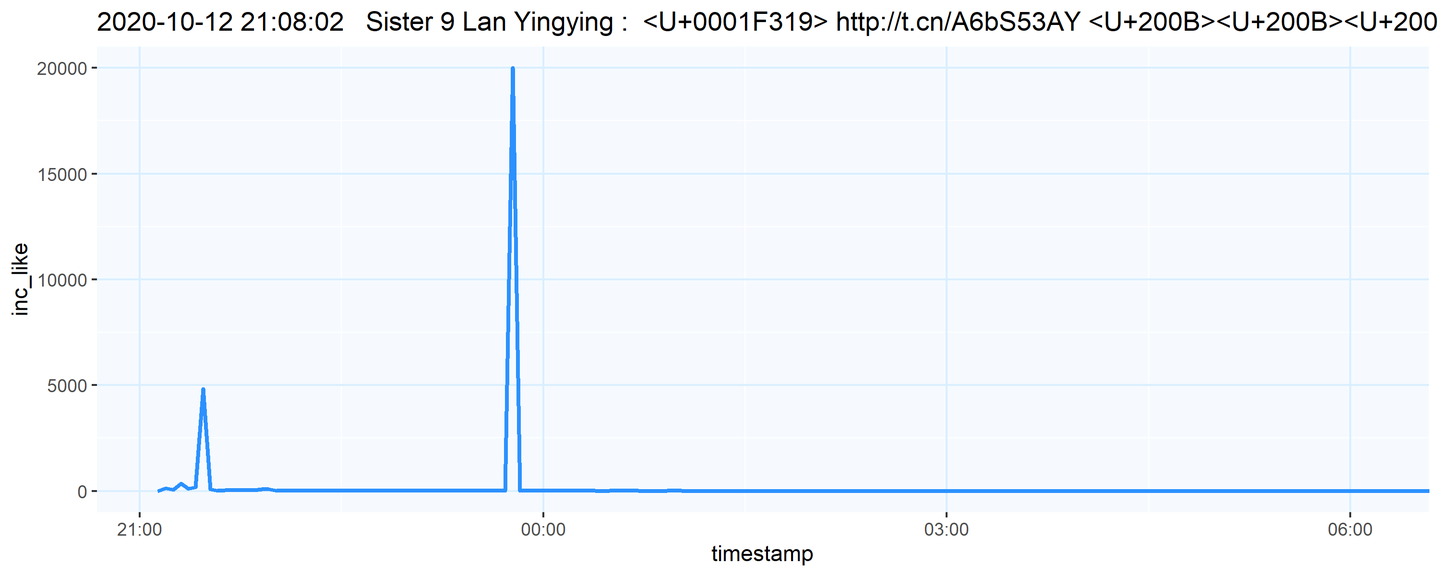• 内容不可能火到上热搜，所以热搜榜没有影响；
• 机器人水军要去主动联系还要排期，因此一时半会也没法到位；
• 粉丝数据组轮博刷评论，也要去群里和超话先布置任务什么的，因此也没法这么快就进来。

## 如何在R中实现微博爬虫

（对技术细节不感兴趣的观众朋友们，你们可以划过这部分，直接看下一个大标题“爬虫监视结果：娱乐圈几乎人均发水，区别只在多少”）

• 找到要爬取的具体网址

``````URLs = 'https://m.weibo.cn/api/container/getIndex?type=uid&value=1757744065&containerid=1076031757744065'
``````

``````### require a package to format json file from weibo
require(jsonlite)

### read json file for weibo lists

### format the json file fetched
json=prettify(json)
writeLines(json,'D:/desktop/weibocrawler/json.txt')
``````

``````### separate the 10 weibos
starts=grep('                "card_type": 9,',page)
ends=grep('                "show_type": 0',page)
``````

• 将取回的json中含有的数据提取出来

``````#### create the data matrix for saving data: weibo ID, timestamp, repost, comment, like
topwb=length(grep('"text": "置顶",',page))!=0
db=matrix(nrow = length(starts),ncol = 8)
if (topwb) {db=matrix(nrow = length(starts)-1,ncol = 8)}
colnames(db)=c('weibo_ID', 'weibo_text', 'timestamp', 'repost', 'comment', 'like', 'isRepost', 'fromChaoHua')

#### fill in the data
for (i in 1:length(starts)) {
weibo=page[starts[i]:ends[i]]

# remove pinned weibo
if (length(grep('"text": "置顶",',weibo))!=0) {next}

# correct i for skipped weibos
rowdb=length(db[,1])-(length(starts)-i)

# get attributes
wbid=gsub('                    "bid": "','',weibo[grep('                    "bid": "',weibo)])
wbid=gsub(',|"|\\s','',wbid)
wbtext=gsub('                    "raw_text": "','',weibo[grep('"raw_text": "',weibo)])
wbtext=gsub('",','',wbtext)
repo=gsub("[^0-9]",'',weibo[grep('"reposts_count": ',weibo)])
like=gsub("[^0-9]",'',weibo[grep('"attitudes_count": ',weibo)])
if (length(grep('                        "retweeted": 1,',weibo))>0) {repost=1} else {repost=0}
if (grepl('超话',weibo[grep('                   "source": ',weibo)])) {fromch=1} else {fromch=0}

# if a weibo is a repost, keep only the data from the user being observed
db[rowdb,1]=as.character(wbid[length(wbid)])
db[rowdb,2]=wbtext[length(wbtext)]
db[rowdb,3]=as.numeric(Sys.time())
db[rowdb,4]=repo[length(repo)]
db[rowdb,5]=comment[length(comment)]
db[rowdb,6]=like[length(like)]
db[rowdb,7]=repost
db[rowdb,8]=fromch
}
``````

``````wbwatcher = function(jsonURL) {
<.........>
return(db)
}
``````

• 自动监视自动存数据

``````while (Sys.time()<"2020-11-10 23:59:59 CEST") { # define stop time
for (i in 1:length(urltable\$URLs)) {
URL=urltable\$URLs[i]
if (length(grep('m.weibo.cn/statuses/show?id=',URL,fixed = T))!=0) {
try({                                        # use try() to prevent breaks from errors caused by network failure
db=wbpostwatcher(URL)
})
if (!is.na(db[1,1])) {
print(paste('Success!', 'Checked', urltable\$names[i], ' at',Sys.time()))
}
}
else {
try({
db=wbwatcher(URL)   # another similar function, used to watch a single weibo only
})
if (!is.na(db[1,1])) {
print(paste('Success!', 'Checked', urltable\$names[i], ' at',Sys.time()))
}
}
db=as.table(db)
if (!is.na(db[1,1])) {
db=cbind(rep(urltable\$names[i],length(db[,1])),db)
colnames(db)='name'
write.table(db, "D:/desktop/weibocrawler/db_sisters.csv", sep = ",", col.names = !file.exists("D:/desktop/weibocrawler/db_sisters.csv"), row.names = F, append = T)
}
else {print(paste('Error! at',Sys.time()))}
Sys.sleep(5)     # Pause query to prevent being banned by weibo
}
}
``````